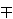### Alan Filipin and Yasutsugu Fujita

Faculty of Civil Engineering, University of Zagreb, Fra Andrije Kačića-Miošića 26, 10 000 Zagreb, Croatia

Department of Mathematics, College of Industrial Technology, Nihon University, 2-11-1 Shin-ei, Narashino, Chiba, Japan
e-mail: fujita.yasutsugu@nihon-u.ac.jp

Abstract.   Let n be a nonzero integer. A set of m distinct positive integers is called a D(n)-m-tuple if the product of any two of them increased by n is a perfect square. Let k be a prime number. In this paper we prove that the D(-k2)-triple {1,k2+1,k2+4} cannot be extended to a D(-k2)-quadruple if k≠3. And for k=3 we prove that if the set {1,10,13,d} is a D(-9)-quadruple, then d=45.

2000 Mathematics Subject Classification.   11D09, 11J68.

Key words and phrases.   Diophantine tuples, simultaneous Diophantine equations.

Full text (PDF) (access from subscribing institutions only)

DOI: 10.3336/gm.46.2.03

References:

1. A. Baker and G. Wüstholz, Logarithmic forms and group varieties, J. Reine Angew. Math. 442 (1993), 19-62.
MathSciNet     CrossRef

2. M. A. Bennett, On the number of solutions of simultaneous Pell equations, J. Reine Angew. Math. 498 (1998), 173-199.
MathSciNet     CrossRef

3. M. A. Bennett, M. Cipu, M. Mignotte and R. Okazaki, On the number of solutions of simultaneous Pell equations II, Acta Arith. 122 (2006), 407-417.
MathSciNet     CrossRef

4. E. Brown, Sets in which xy+k is always a square, Math. Comp. 45 (1985), 613-620.
MathSciNet     CrossRef

5. A. Dujella, Generalization of a problem of Diophantus, Acta Arith. 65 (1993), 15-27.
MathSciNet

6. A. Dujella, Complete solution of a family of simultaneous Pellian equations, Acta Math. Inform. Univ. Ostraviensis 6 (1998), 59-67.
MathSciNet

7. A. Dujella, An absolute bound for the size of Diophantine m-tuples, J. Number Theory 89 (2001), 126-150.
MathSciNet     CrossRef

8. A. Dujella, There are only finitely many Diophantine quintuples, J. Reine Angew. Math. 566 (2004), 183-214.
MathSciNet     CrossRef

9. A. Dujella, A. Filipin and C. Fuchs, Effective solution of the D(-1)-quadruple conjecture, Acta Arith. 128 (2007), 319-338.
MathSciNet     CrossRef

10. A. Dujella and C. Fuchs, Complete solution of a problem of Diophantus and Euler, J. London Math. Soc. 71 (2005), 33-52.
MathSciNet     CrossRef

11. A. Dujella and A. Pethö, A generalization of a theorem of Baker and Davenport, Quart. J. Math. Oxford Ser. (2) 49 (1998), 291-306.
MathSciNet     CrossRef

12. Y. Fujita, The non-extensibility of D(4k)-triples {1,4k(k-1),4k2+1} with |k| prime, Glas. Mat. Ser. III 41 (2006), 205-216.
MathSciNet     CrossRef

13. Y. Fujita, Extensions of the D(k2)-triples {k2,k2±1,4k2±1}, Period. Math. Hungar. 59 (2009), 81-98.
MathSciNet     CrossRef

14. Y. Fujita, The number of Diophantine quintuples, Glas. Mat. Ser. III 45 (2010), 15-29.
MathSciNet     CrossRef

15. Y. Fujita and A Togbé, The extension of the D(-k2)-pair {k2,k2+1}, Period. Math. Hungar., to appear.

16. R. A. Mollin, Quadratics, CRC Press, Boca Ranton, 1996.
MathSciNet

17. J. E. Shockley, Introduction to Number Theory, Holt, Rinehart and Winston, 1967.
MathSciNet